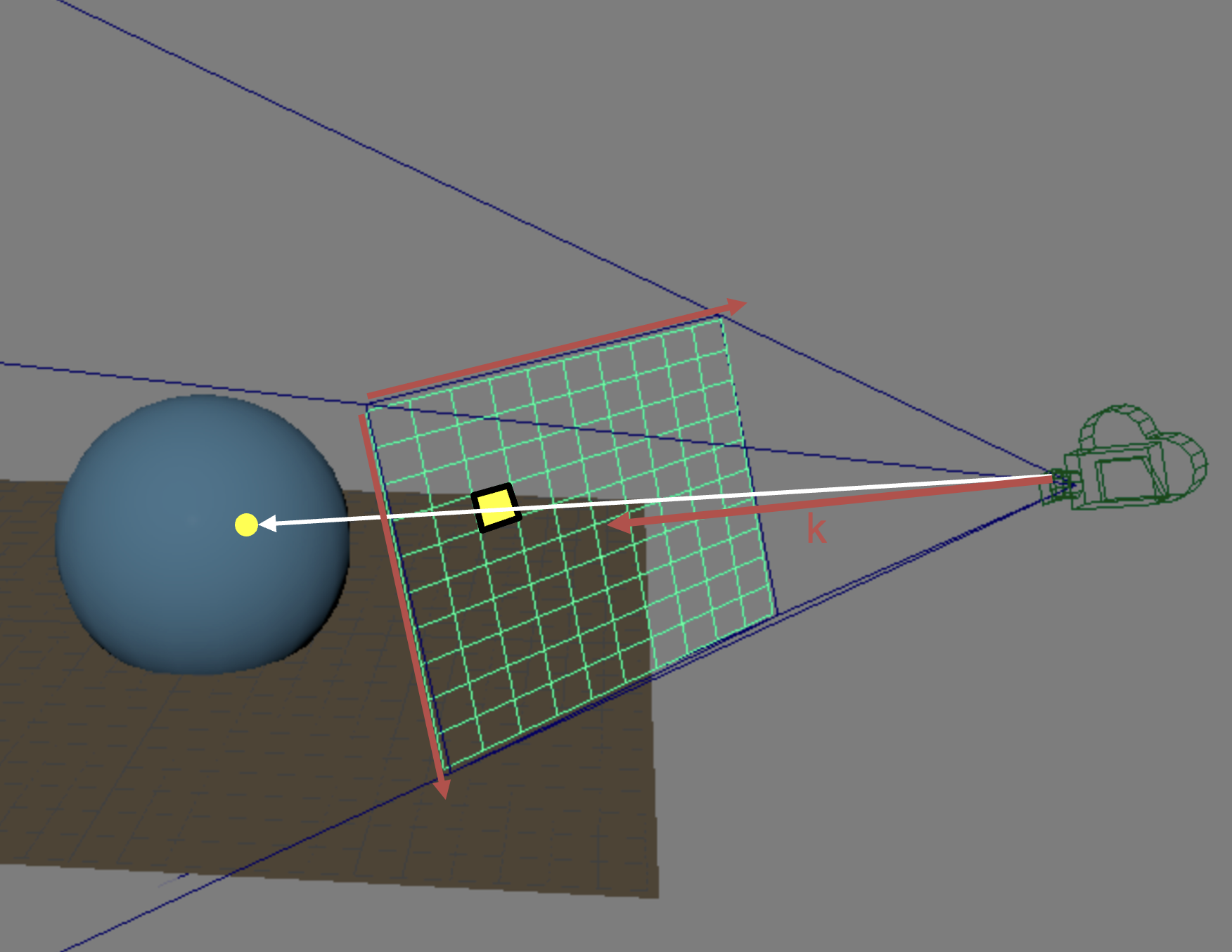# Project 3: Intersect (Algo Answers)

## Cameras

When implementing ray tracing, you will often need to transform points between different coordinate spaces, e.g. between the world and camera spaces.

Remember that a camera's view matrix describes a transformation which takes points from world space to camera space.

Suppose you are given the position, look vector, and up vector of a virtual camera. How would you use this information to compute the camera's view matrix? If you prefer, you may describe what should be computed instead of writing mathematical equations.

The basis vectors of the camera's coordinate space, , , and , can be computed based on the and vectors:

From these, we can construct the rotation matrix :

Since we're given the camera's position, , we can also construct the translation matrix :

Finally, we can compose and to obtain :

Explanation:

Refer to our lecture slides on camera models & viewing transforms or your lab 4 code.

## Generating Rays

### Pixels In Space

Recall that the view plane describes an imaginary 2D plane, perpendicular to the camera's look vector, which is some distance away from the camera. Here's a diagram:Figure 1: An example of a view plane with one pixel highlighted. A ray is shot through that pixel, and intersects a sphere in the scene.

Now, suppose you are given the following information about a specific pixel in an image:

• : image width, in pixels
• : image height, in pixels
• : our pixel's row index
• : our pixel's column index
• : depth of the view plane from the camera position
• : height view angle
• : width view angle

Please calculate the 3D point on the view plane, (x, y, z), in camera space, which corresponds with this pixel.

For the purpose of this algo, we use to express the depth of the view plane from camera position. However, this value is arbitrary—in your implementation, feel free to set it to . Why do you think that is the case? (Not an algo question)

As always, please assume that the pixel (0, 0) corresponds to the top left pixel of the image.

Explanation:

and give the relative coordinates of x and y on the unit view plane. Next, we just need to figure out the actual size of the view plane, which can be computed via height angle and the depth of the view plane.

Hence, the , are:

### Rays

We would like to shoot a ray from our camera, located at in world space, through a particular pixel's point on the view plane, in camera space. See Figure 1 for an example.

Please write an expression for this ray in the form , where is the origin of the ray, and is a vector in the direction it's being shot at. Both and must be in world space, so you're going to also need , the camera's view matrix.

Explanation:

We compute our pixel's point on the view plane in world space as . We can then obtain the ray's direction, , by subtracting the camera's position in world space.

Meanwhile, the ray's origin, , is simply the camera's position in world space. With this information, we can easily express our ray as above.

Note: you can choose not to normalize . We chose to do so as we like unit-length direction vectors.

## Implicit Shape Primitives

In lecture, we derived implicit equations for spheres and cylinders to perform intersection tests. In this question, you will attempt to do the same with a cone.

Begin by writing out the implicit equations for a cone with the following parameters:

• Radius = 0.5 at the flat base.
• Height = 1
• Origin is halfway along the height of the cone.

You will have to write two implicit equations, one for the conical top and one for the flat base. Each should be of the form , and each should have zero or more boundary conditions (e.g. ).

Next, you will solve these implicit equations for their intersection points with some arbitrary ray . You will have to find values of such that satisfies our implicit equations.

Please find the values of at the points along the ray which intersect with the cone. Give in terms of and , then state for what conditions this solution is valid.

Example of Boundary Checking:

• solves for the flat base, but it is not a valid solution.

Your final answer might be pretty long. Feel free to use sub-expressions!

Conical top

Solution is valid if .

Flat base

Solution is valid if .

Explanation:

Conical top

The radius of the cone at some is given by this expression:

Where and is the slope, which can easily be found to be in our case.

Hence, the equation of the conical top is:

We can express the intersection point in terms of :

By solving this equation, we can obtain the answer above.

Flat base

The equation for the flat base is:

This follows from the equation for a circle.

If you got this question correct, you got an extra point on the algo assignment!

## Transforming Object Normals

In the first part of the ray project, you will need to compute the lighting equation using the world space normal at the intersection point. From the previous question you may notice we have only computed the object space postion and normal. Thus we are going to have to transform the intersection data from object space to world space.

For positions, you already know how to do this (think back to lab 4!). However, transforming normals is not as simple as multiplying by the model matrix.

Write down the equation for the world space normal vector, , in terms of the following...

• : the normal vector in object-space.
• : the object's model matrix

, where is the upper-left 3x3 portion of the object's modeling transformation matrix, and is the inverse of its transpose.